Home > CC2 > Chapter 8 > Lesson 8.3.1 > Problem8-61

8-61.
1. Write an equation that represents each diagram below and find the value of the variable. Homework Help ✎

1.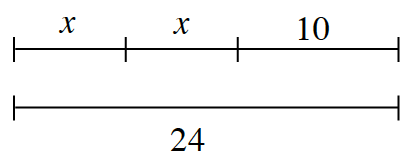2.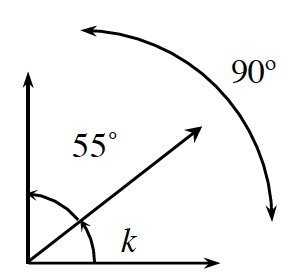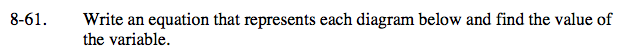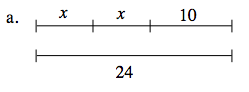How are the two lines related?
How will this help you write an equation?

Because the two lines are equal, you can write the equation:
x + x + 10 = 24

Combine like terms
2x + 10 = 24

Then solve for x:
2x = 14
x = 7

x = 7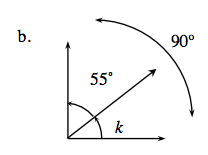Use the same method as part (a).